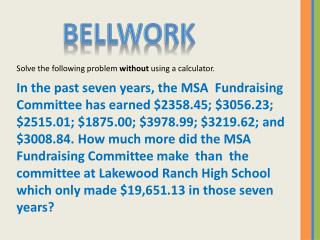DownloadDownload PresentationBellwork

# Bellwork

Download Presentation## Bellwork

- - - - - - - - - - - - - - - - - - - - - - - - - - - E N D - - - - - - - - - - - - - - - - - - - - - - - - - - -
##### Presentation Transcript

1. Bellwork Solve the following problem without using a calculator. In the past seven years, the MSA Fundraising Committee has earned \$2358.45; \$3056.23; \$2515.01; \$1875.00; \$3978.99; \$3219.62; and \$3008.84. How much more did the MSA Fundraising Committee make than the committee at Lakewood Ranch High School which only made \$19,651.13 in those seven years?

2. Unit 1.2 Notes Multiplying & Dividing Decimals

3. Multiply Decimals Decimals are multiplied as if they were whole numbers. (It is not necessary to line up the decimal points.) Do you remember how to place the decimal point in your final answer? 4.5 x 7.1 = 31.95 8.789 x 4 = 35.156 .08 x .09 = .0072

4. Multiply Decimals The decimal point in the answer is then found by using the following steps. STEP 1: Count the total number of digits to the right of the decimal point in each of the numbers being multiplied. STEP 2: In the answer, count from right to left the number of places found in STEP 1 AND WRITE THE DECIMAL POINT. It may be necessary to attach zeros to the left of the answer in order to correctly place the decimal point. 4.5 x 7.1 = 31.95  1 + 1 = 2 8.789 x 4 = 35.156  3 + 0 = 3 .08 x .09 = .0072  2 + 2 = 4

5. Examples a) 8.34 x 4.2 b) .032 x .07 a) 8.34 x 4.2 2 decimals 3 decimals b) .032 x .07 1 decimal 2 decimals 1668 .00224 5 decimals 33360 35.028 3 decimals

6. Your Turn a) 6.7 x 4.32 b) .086 x .05 Answers 28.944 .0043

7. Gross Pay Gross pay = Number of hours worked x Pay per hour Find the gross pay of a SUBWAY employee working 31.5 hours at a rate of \$8.65 per hour. Answer: \$272.48

8. Divide Decimals Dividing terms: Quotient, Dividend, Divisor Quotient 7.31 3 )21.93 Divisor Dividend Place a decimal point directly above the dividend’s decimal point.

9. Divide Decimals Example 1: Sometimes it is necessary to place zeros after the decimal point in the dividend. Why? 2.84 5)14.2 5)14.20 Does attaching zeros after the decimal point change the value of the dividend? No.

10. Divide Decimals Example 2: A remainder of 0 might not be obtained even though extra zeros are placed after the dividend. 1.5666 3 )4.7000 Round to the nearest thousandth by carrying out the division to four places and then rounding to three places.

11. Divide Decimals Example 3: To divide by a decimal, first convert the divisor to a whole number. 92.0 . . .3 )27.6 Round to the nearest thousandth by carrying out the division to four places and then rounding to three places.

12. Your Turn a) 17.6 ÷ .25 b) 5 ÷ .42 Answers 70.4 11.905